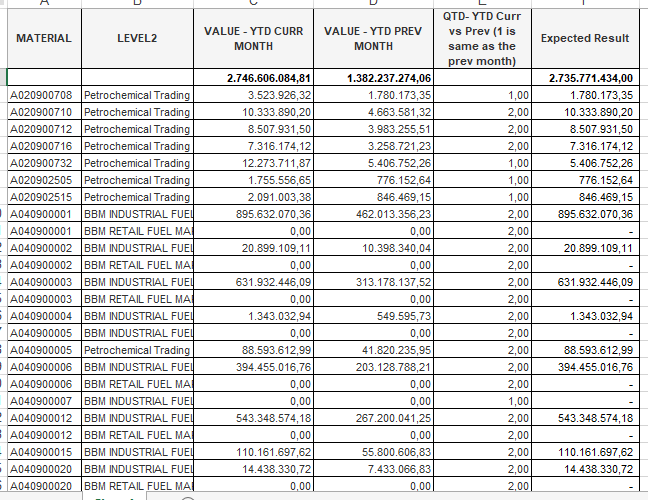# QlikView App Development

Discussion Board for collaboration related to QlikView App Development.

Announcements
Leverage your QlikView investment to modernize BI – see how! Join Group
cancel
Showing results for
Did you mean:
HighlightedPartner

## [ASK] IF-ELSE Condition inside Aggr

Dear QlikView Master,

I have a client that required sum value based on year-to-date quantity condition.

So if Quantity YTD Current Month is not the same as YTD Previous Month, then sum YTD Current Month Value , else sum YTD Previous Month Value.

Currently in my straight table chart i use if-else condition like below.

The formula condition is similar to :

if([Sum Qty YTD Cur Month]=[Sum Qty YTD Prev Month],[Sum Amount YTD Current Month],0)

+

if([Sum Qty YTD Cur Month]=[Sum Qty YTD Prev Month],0,[Sum Amount YTD Current Month])

If i use that formula, it shows correct result if i use 'TOtal Mode : Sum of Rows'.

But if i use pivot table, it doesn't show correct result, only [Sum Amount YTD Current Month]

Based on my above description, is there any posible way to achieve that?

Below is my excel simulation to give more clearer picture.Many thank you,

Best Regards,

Tags (1)
HighlightedMVP

## Re: [ASK] IF-ELSE Condition inside Aggr

You need to use Sum(Aggr()) to get this right

Sum(Aggr(

if([Sum Qty YTD Cur Month]=[Sum Qty YTD Prev Month],[Sum Amount YTD Current Month],0)

+

if([Sum Qty YTD Cur Month]=[Sum Qty YTD Prev Month],0,[Sum Amount YTD Current Month])

,MATERIAL, LEVEL2))